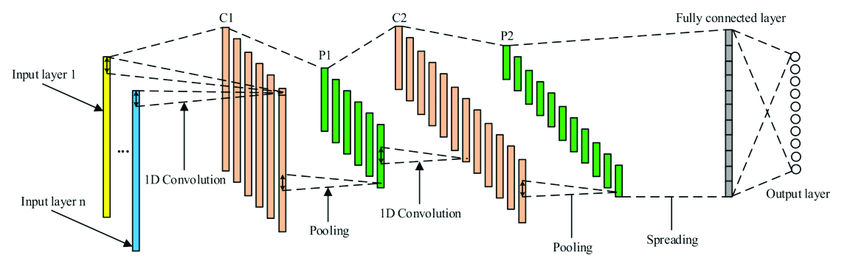# Developing With Keras Functional API

Source: Deep Learning on Medium# Developing With Keras Functional API

Keras sequential api is a popular model for creating any deep learning model. However the model comes with the assumption that it would take one input and would have only one output. Models with this structure is of no use when it is required to have more than one inputs and output as well. Also some deep learning networks might have multiple internal branches(eg. inception module) which is quite different from the traditional sequential model. To deal with such architectures the functional api of keras is quite handy.

In the functional api we consider the layers as function which take tensor as input and also output a tensor as well.

## Syntax Of Functional API:

Unlike the sequential api we have to provide the shape of the input in case of the functional api. So initial step is to provide the shape of the input.

`from keras.layers import Inputfrom keras.models import Modelx = Input(shape=(32,))`

In the above code block we have taken a tensor as input.

`y=layers.Dense(16,activation= ‘relu’)(x)y=layers.Dense(16,activation= ‘relu’)(y)z=layers.Dense(10,activation= ‘softmax’) (x)`

Here we have built our simple deep learning model where we can see even layer is used as a function. And in the end it produces a tensor as an output.

`model=Model(x,z)`

In the final step we have converted our input and output tensor into a model the model object. The final output tensor z is obtained by transforming the input tensor x. In case we try to provide the model any shape apart from the defined one it would lead to runtime error.

We can form directed-acyclic graph using the keras functional api. Here a key thing to note that we can only for acyclic graph as cyclic graph can have condition where a tensor x can become input to another layer that produced x,which is logically incorrect.

# Inception Model Using Functional API:

The above diagram shows a basic inception architecture. The model has got a very complex architecture which we can not code with the basic sequential api. Here we can see branches of independent network. Without digging much into theoretical aspect of an inception module let us look at the coding part of it.

`from keras import layerspart_a = layers.Conv2D(128, 1,activation=’relu’, strides=2)(x)part_b = layers.Conv2D(128, 1, activation=’relu’)(x)part_b = layers.Conv2D(128, 3, activation=’relu’, strides=2)(part_b)part_c = layers.Conv2D2D(128,1, strides=2)(x)part_c = layers.Conv2D(128, 5, activation=’relu’)(part_c)part_d = layers.MaxPooling(128, 3, activation=’relu’)(x)part_d = layers.Conv2D(128, 1, activation=’relu’)(part_d)output = layers.concatenate([part_a, part_b, part_c, part_d], axis=-1)`

Here each part refers to a branch of an inception module. Here as we can see x is an input from the previous layer. The part_a refers to the first branch which has 128 number of 1*1 kernels. In the second branch part_b we have an initial 1*1 convolution and then a 3*3 convolution. The initial 1*1 convolution takes input from the previous layer (i.e x) whereas the second 3*3 takes input from the output of the former i.e (output of first layer of part_b). Similarly we can understand the code for part_c and part_d as well.In the final step we have concatenated output of all the four branches.

Here is a keypoint that should be noticed that we have kept stride of 2 for all the layers. This is done so that in the final concatenation we can have all the outputs of same shape else it would show error.

# Residual Connection Using Functional API:

Residual connection helps in tackling the problem of vanishing gradient problem of longer networks. It directly provides out of a previous layer to a later layer directly.

`from keras import layers,Inputx=Input(shape=(None,))y=layers.Conv2D(128, 3, activation=’relu’, padding=’same’)(x)y=layers.Conv2D(128, 3, activation=’relu’, padding=’same’)(y)y=layers.Conv2D(128, 3, activation=’relu’, padding=’same’)(y)y=layers.add([y, x])`

The above code is self-explanatory. The key thing here is that we are using the input of the earlier layer as an input to the later layer y. In case any discrepancy happens with the shape we can make use of 1*1 convolution to get the required shape.

# Use Of Models As Layers:

We can also use models as we use layers.For example let us say we have got a trained model named mod. And we want to use the model mod similarly we use the layer instance.For an example consider the below point

`y=mod(x)`

Here mod is an already trained model which we are using for producing output for an intermediate layer. We are getting the output tensor y when we provide x as an intermediate tensor input.

This article showed the power of functional api which enables us to create any kind of complex model architecture which is beyond the reach of a basic sequential model.